Geometry Set A 2014-2015 SSC (English Medium) 10th Standard Board Exam Question Paper Solution

Geometry [Set A]
Date: March 2015
Duration: 2h

 1 | Solve any five sub-questions :
 1.1

In the following figure seg AB ⊥ seg BC, seg DC ⊥ seg BC. If AB = 2 and DC = 3, find (A(triangleABC))/(A(triangleDCB))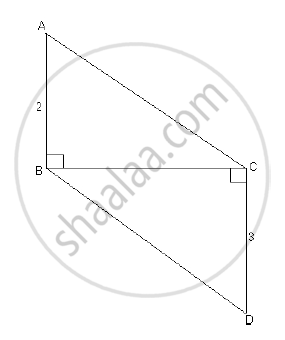Concept: Properties of Ratios of Areas of Two Triangles
Chapter: [0.01] Similarity
 1.2

Find the slope and y-intercept of the line y = -2x + 3.

Concept: Intercepts Made by a Line
Chapter: [0.04] Co-ordinate Geometry
 1.3

In the following figure, in ΔABC, BC = 1, AC = 2, ∠B = 90°. Find the value of sin θ.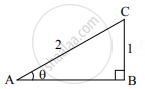Concept: Heights and Distances
Chapter: [0.06] Trigonometry
 1.4

Find the diagonal of a square whose side is 10 cm.

Concept: Circumference of a Circle
Chapter: [0.07] Mensuration [0.07] Mensuration
 1.5

The volume of a cube is 1000 cm3. Find the side of a cube.

Concept: Surface Area of a Combination of Solids
Chapter: [0.07] Mensuration
 1.6

If two circles with radii 5 cm and 3 cm respectively touch internally, find the distance between their centres.

Concept: Touching Circles
Chapter: [0.03] Circle
 2 | Solve any four sub-questions :
 2.1

If sin θ =7/25, where θ is an acute angle, find the value of cos θ.

Concept: Trigonometric Ratios of Complementary Angles
Chapter: [0.06] Trigonometry
 2.2

Draw ∠ABC of measure 120° and bisect it.

Concept: Basic Geometric Constructions
Chapter: [0.05] Geometric Constructions
 2.3

Find the slope of the line passing through the points M(4,0) and N(-2,-3).

Concept: Slope of a Line
Chapter: [0.04] Co-ordinate Geometry
 2.4

Find the area of the sector whose arc length and radius are 14 cm and 6 cm respectively.

Concept: Areas of Sector and Segment of a Circle
Chapter: [0.07] Mensuration
 2.5

In the following figure, in Δ PQR, seg RS is the bisector of ∠PRQ.

PS = 11, SQ = 12, PR = 22. Find QR.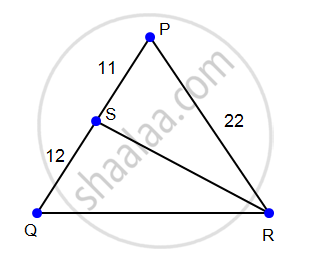Concept: Similarity of Triangles
Chapter: [0.01] Similarity
 2.6

In the following figure, if m(arc DXE) = 120° and m(arc AYC) = 60°. Find ∠DBE.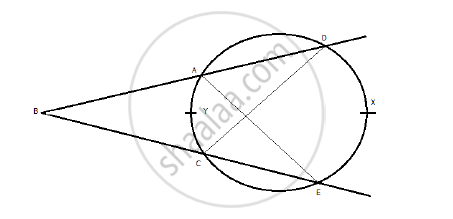Concept: Areas of Sector and Segment of a Circle
Chapter: [0.07] Mensuration
 3 | Solve any three sub-questions :
 3.1

In the following figure, Q is the centre of a circle and PM, PN are tangent segments to the circle. If ∠MPN = 60°, find ∠MQN.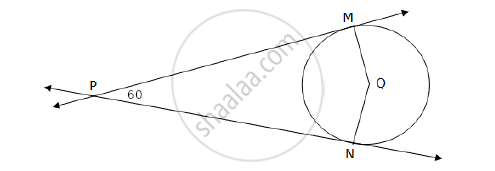Concept: Number of Tangents from a Point on a Circle
Chapter: [0.03] Circle [0.03] Circle
 3.2

Draw the tangents to the circle from the point L with radius 3 cm. Point ‘L’ is at a distance 8 cm from the centre ‘M’.

Concept: Construction of Tangents to a Circle
Chapter: [0.05] Geometric Constructions
 3.3

The ratio of the areas of two triangles with the common base is 4 : 3. Height of the larger triangle is 2 cm, then find the corresponding height of the smaller triangle.

Concept: Properties of Ratios of Areas of Two Triangles
Chapter: [0.01] Similarity
 3.4

Two buildings are in front of each other on either side of a road of width 10 metres. From the top of the first building which is 20 metres high, the angle of elevation to the top of the second is 45°. What is the height of the second building?

Concept: Heights and Distances
Chapter: [0.06] Trigonometry
 3.5

Find the volume and surface area of a sphere of radius 8.4 cm.

(pi=22/7)

Concept: Surface Area of a Combination of Solids
Chapter: [0.07] Mensuration
 4
 4.1

Prove that ‘the opposite angles of a cyclic quadrilateral are supplementary’.

Chapter: [0.03] Circle
 4.2

Prove that sin6θ + cos6θ = 1 – 3 sin2θ. cos2θ.

Concept: Trigonometric Identities
Chapter: [0.06] Trigonometry
 4.3

A test tube has diameter 20 mm and height is 15 cm. The lower portion is a hemisphere. Find the capacity of the test tube. (π = 3.14)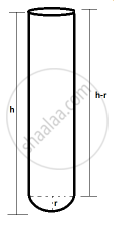Concept: Surface Area of a Combination of Solids
Chapter: [0.07] Mensuration
 5 | Solve any two sub-questions :
 5.1

Prove that the angle bisector of a triangle divides the side opposite to the angle in the ratio of the remaining sides.

Concept: Similarity of Triangles
Chapter: [0.01] Similarity
 5.2

Write down the equation of a line whose slope is 3/2 and which passes through point P, where P divides the line segment AB joining A(-2, 6) and B(3, -4) in the ratio 2 : 3.

Concept: Division of a Line Segment
Chapter: [0.04] Co-ordinate Geometry [0.05] Geometric Constructions
 5.3

ΔRST ~ ΔUAY, In ΔRST, RS = 6 cm, ∠S = 50°, ST = 7.5 cm. The corresponding sides of ΔRST and ΔUAY are in the ratio 5 : 4. Construct ΔUAY.

Concept: Division of a Line Segment
Chapter: [0.04] Co-ordinate Geometry [0.05] Geometric Constructions

Request Question Paper

If you dont find a question paper, kindly write to us

View All Requests

Submit Question Paper

Help us maintain new question papers on Shaalaa.com, so we can continue to help students

only jpg, png and pdf files

Maharashtra State Board previous year question papers 10th Standard Board Exam Geometry with solutions 2014 - 2015

Maharashtra State Board 10th Standard Board Exam Geometry question paper solution is key to score more marks in final exams. Students who have used our past year paper solution have significantly improved in speed and boosted their confidence to solve any question in the examination. Our Maharashtra State Board 10th Standard Board Exam Geometry question paper 2015 serve as a catalyst to prepare for your Geometry board examination.
Previous year Question paper for Maharashtra State Board 10th Standard Board Exam Geometry-2015 is solved by experts. Solved question papers gives you the chance to check yourself after your mock test.
By referring the question paper Solutions for Geometry, you can scale your preparation level and work on your weak areas. It will also help the candidates in developing the time-management skills. Practice makes perfect, and there is no better way to practice than to attempt previous year question paper solutions of Maharashtra State Board 10th Standard Board Exam.

How Maharashtra State Board 10th Standard Board Exam Question Paper solutions Help Students ?
• Question paper solutions for Geometry will helps students to prepare for exam.
• Question paper with answer will boost students confidence in exam time and also give you an idea About the important questions and topics to be prepared for the board exam.
• For finding solution of question papers no need to refer so multiple sources like textbook or guides.